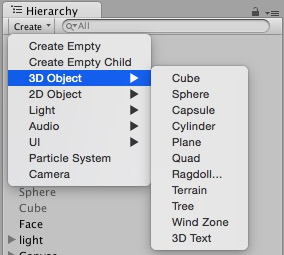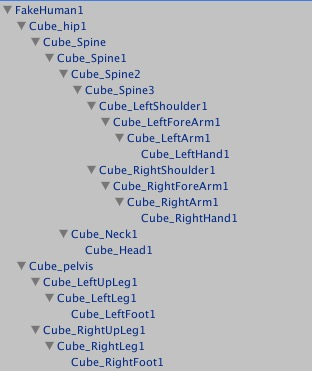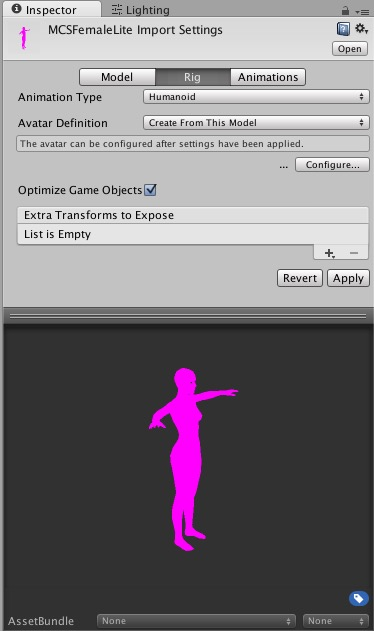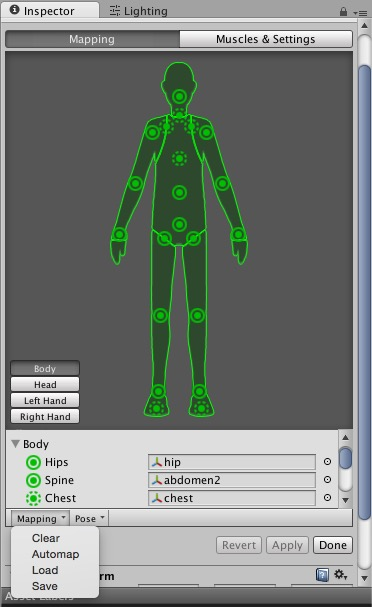## Introduction

Controlling Objects in Unity with The Captury

- Cover the OSC[[OSC|Open Sound Control]] syntax and how to get nodes as matrix / vector, absolute and relative
- How to create objects in Unity and arrange them so they represent the nodes

- How to animate these Objects

## Rotation matrix, Quaternion

At first we should understand some concepts.There are three ways to represent rotation that is rotation matrix, quaternion and Euler, theCaputry uses rotation matrix, Unity uses Quaternion and Euler. This tutorial focus on Rotation matrix, Quaternion.
4×4 Rotation matrix
TheCaputry uses it to represent rotation. Unity had only provided a 4 × 4 matrix class Matrix4x4, it contains transform T, rotation R and scaling information. You can refer to .

Any vector can be the rotation axis;
1. In fact, to rotate a object only need 4 values ,a vector(x,y,z) and a angle, but the matrix has 16 elements; 2. It will increase the amount of calculation when doing multiplication operation and resultin some waste of space and time;

Quaternion
Quaternions are used to represent rotations. Mostly you can use Quaternion AngleAxis(float angle, Vector3 axis) to create a quaternion.Unity internally uses Quaternions to represent all rotations. you can refer to:

1. They are compact, don't suffer from gimbal lock and can easily be interpolated;
2. It only need a 4-dimensional quaternion through the origin then can execute arbitrary rotation. In some cases it has higher efficiency than rotation matrix;
3. It can provide a smooth interpolation;

1.Compare to Euler it has one more dimension, so it will be difficult to understand.

## Conversion between Rotation matrix and Quaternion

We might need to convert rotation matrix to quaternion depend on your project needs after we get data from theCaptury in matrix format.There i present two ways to convert there two.

Convert quaternion to 4×4 Rotation matrix.
```Quaternion q = Quaternion.LookRotation(new Vector3(0,0.5,1));
Matrix4x4 rot = new Matrix4x4();
rot.SetTRS(new Vector3(0,0,0),q,new Vector3(1,1,1));```
Convert 4×4 Rotation matrix to quaternion.
```Matrix4x4 rot = new Matrix4x4();
rot.SetTRS(new Vector3(0,0,0),q,new Vector3(1,1,1));  //It’s the same “q” that used above
Vector4 vy = rot.GetColumn(1);
Vector4 vz = rot.GetColumn(2);
Quaternion newQ = Quaternion.LookRotation(new Vector3(vz.x,vz.y,vz.z),new Vector3(vy.x,vy.y,vy.z));```

Other conversion:
Euler to Quaternion
Quaternion To Euler
AngleAxis to Quaternion
Quaternion to AngleAxis

## Cover the OSC[[OSC|Open Sound Control]] syntax and how to get nodes as matrix / vector, absolute and relative

Cover the OSC[[OSC|Open Sound Control]] syntax and get nodes as matrix / vector
With OSC[[OSC|Open Sound Control]] you can subscribe to the following: 1. subscribe to a bone position,rotation as vector in world coordinates for positions:

/subscribe/<name of person1>/blender/@/vector

2. subscribe to a bone position,rotation as vector in relative coordinates for positions:

/subscribe/<name of person1>/blender/@/upper/vector

3. subscribe to a bone position,rotation as matrix in world coordinates:

/subscribe/<name of person1>/blender/@/matrix

4. subscribe to a bone position,rotation as matrix in relative coordinates:

/subscribe/<name of person1>/blender/@/upper/matrix

You can use transform.localPosition to get position of the transform relative to the parent transform.

## How to create objects in Unity and arrange them so they represent the nodes

1. Create a gameobject or you can import your own model into Unity2. Arrange the cube in a certain hierarchyor you can import your model then automap it.## How to animate these Objects

```	msg = handler.StringToOscMessage("/subscribe/fiona/blender/Root/vector 50.0 0.0 20.0");
handler.Send(msg);```
2. Getting data;
```switch (msgAddress){
case "/Fiona_fit/blender/Root/vector":
hip = values;
hip = values;
hip = values;
break;}```
3. Giving the data to the object.
```	var transHip = GameObject.Find("Cube_hip1");
transHip.transform.position = Vector3(hip, -hip, hip);```

4. Video In this video i use cube to replace every bone.